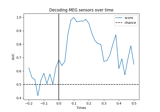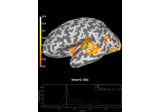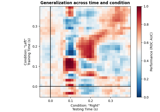# mne.decoding.SlidingEstimator#

class mne.decoding.SlidingEstimator(base_estimator, scoring=None, n_jobs=None, *, position=0, verbose=None)[source]#

Search Light.

Fit, predict and score a series of models to each subset of the dataset along the last dimension. Each entry in the last dimension is referred to as a task.

Parameters:
base_estimatorobject

The base estimator to iteratively fit on a subset of the dataset.

scoring

Score function (or loss function) with signature `score_func(y, y_pred, **kwargs)`. Note that the “predict” method is automatically identified if scoring is a string (e.g. `scoring='roc_auc'` calls `predict_proba`), but is not automatically set if `scoring` is a callable (e.g. `scoring=sklearn.metrics.roc_auc_score`).

n_jobs

The number of jobs to run in parallel. If `-1`, it is set to the number of CPU cores. Requires the `joblib` package. `None` (default) is a marker for ‘unset’ that will be interpreted as `n_jobs=1` (sequential execution) unless the call is performed under a `joblib.parallel_backend()` context manager that sets another value for `n_jobs`.

position`int`

The position for the progress bar.

verbose

Control verbosity of the logging output. If `None`, use the default verbosity level. See the logging documentation and `mne.verbose()` for details. Should only be passed as a keyword argument.

Attributes:

List of fitted scikit-learn estimators (one per task).

Methods

 Estimate distances of each data slice to the hyperplanes. `fit`(X, y, **fit_params) Fit a series of independent estimators to the dataset. `fit_transform`(X, y, **fit_params) Fit and transform a series of independent estimators to the dataset. `get_params`([deep]) Get parameters for this estimator. Predict each data slice/task with a series of independent estimators. Predict each data slice with a series of independent estimators. `score`(X, y) Score each estimator on each task. `set_params`(**params) Set the parameters of this estimator. Transform each data slice/task with a series of independent estimators.
decision_function(X)[source]#

Estimate distances of each data slice to the hyperplanes.

Parameters:
X`array`, shape (n_samples, nd_features, n_tasks)

The input samples. For each data slice, the corresponding estimator outputs the distance to the hyperplane, e.g.: `[estimators[ii].decision_function(X[..., ii]) for ii in range(n_estimators)]`. The feature dimension can be multidimensional e.g. X.shape = (n_samples, n_features_1, n_features_2, n_estimators).

Returns:
y_pred`array`, shape (n_samples, n_estimators, n_classes * (n_classes-1) // 2)

Predicted distances for each estimator/data slice.

Notes

This requires base_estimator to have a `decision_function` method.

fit(X, y, **fit_params)[source]#

Fit a series of independent estimators to the dataset.

Parameters:
X`array`, shape (n_samples, nd_features, n_tasks)

The training input samples. For each data slice, a clone estimator is fitted independently. The feature dimension can be multidimensional e.g. X.shape = (n_samples, n_features_1, n_features_2, n_tasks).

y`array`, shape (n_samples,) | (n_samples, n_targets)

The target values.

**fit_params`dict` of `str` -> object

Parameters to pass to the fit method of the estimator.

Returns:
selfobject

Return self.

Examples using `fit`:Decoding (MVPA)

Decoding (MVPA)Decoding source space data

Decoding source space dataDecoding sensor space data with generalization across time and conditions

Decoding sensor space data with generalization across time and conditions
fit_transform(X, y, **fit_params)[source]#

Fit and transform a series of independent estimators to the dataset.

Parameters:
X`array`, shape (n_samples, nd_features, n_tasks)

The training input samples. For each task, a clone estimator is fitted independently. The feature dimension can be multidimensional, e.g.:

```X.shape = (n_samples, n_features_1, n_features_2, n_estimators)
```
y`array`, shape (n_samples,) | (n_samples, n_targets)

The target values.

**fit_params`dict` of `str` -> object

Parameters to pass to the fit method of the estimator.

Returns:
y_pred`array`, shape (n_samples, n_tasks) | (n_samples, n_tasks, n_targets)

The predicted values for each estimator.

get_params(deep=True)[source]#

Get parameters for this estimator.

Parameters:
deep`bool`, optional

If True, will return the parameters for this estimator and contained subobjects that are estimators.

Returns:
params`dict`

Parameter names mapped to their values.

predict(X)[source]#

Predict each data slice/task with a series of independent estimators.

The number of tasks in X should match the number of tasks/estimators given at fit time.

Parameters:
X`array`, shape (n_samples, nd_features, n_tasks)

The input samples. For each data slice, the corresponding estimator makes the sample predictions, e.g.: `[estimators[ii].predict(X[..., ii]) for ii in range(n_estimators)]`. The feature dimension can be multidimensional e.g. X.shape = (n_samples, n_features_1, n_features_2, n_tasks).

Returns:
y_pred`array`, shape (n_samples, n_estimators) | (n_samples, n_tasks, n_targets)

Predicted values for each estimator/data slice.

predict_proba(X)[source]#

Predict each data slice with a series of independent estimators.

The number of tasks in X should match the number of tasks/estimators given at fit time.

Parameters:
X`array`, shape (n_samples, nd_features, n_tasks)

The input samples. For each data slice, the corresponding estimator makes the sample probabilistic predictions, e.g.: `[estimators[ii].predict_proba(X[..., ii]) for ii in range(n_estimators)]`. The feature dimension can be multidimensional e.g. X.shape = (n_samples, n_features_1, n_features_2, n_tasks).

Returns:
y_pred`array`, shape (n_samples, n_tasks, n_classes)

Predicted probabilities for each estimator/data slice/task.

score(X, y)[source]#

Score each estimator on each task.

The number of tasks in X should match the number of tasks/estimators given at fit time, i.e. we need `X.shape[-1] == len(self.estimators_)`.

Parameters:
X`array`, shape (n_samples, nd_features, n_tasks)

The input samples. For each data slice, the corresponding estimator scores the prediction, e.g.: `[estimators[ii].score(X[..., ii], y) for ii in range(n_estimators)]`. The feature dimension can be multidimensional e.g. X.shape = (n_samples, n_features_1, n_features_2, n_tasks).

y`array`, shape (n_samples,) | (n_samples, n_targets)

The target values.

Returns:
score`array`, shape (n_samples, n_estimators)

Examples using `score`:Decoding sensor space data with generalization across time and conditions

Decoding sensor space data with generalization across time and conditions
set_params(**params)[source]#

Set the parameters of this estimator.

The method works on simple estimators as well as on nested objects (such as pipelines). The latter have parameters of the form `<component>__<parameter>` so that it’s possible to update each component of a nested object.

Parameters:
**params`dict`

Parameters.

Returns:
instinstance

The object.

transform(X)[source]#

Transform each data slice/task with a series of independent estimators.

The number of tasks in X should match the number of tasks/estimators given at fit time.

Parameters:
X`array`, shape (n_samples, nd_features, n_tasks)

The input samples. For each data slice/task, the corresponding estimator makes a transformation of the data, e.g. `[estimators[ii].transform(X[..., ii]) for ii in range(n_estimators)]`. The feature dimension can be multidimensional e.g. X.shape = (n_samples, n_features_1, n_features_2, n_tasks).

Returns:
Xt`array`, shape (n_samples, n_estimators)

The transformed values generated by each estimator.

## Examples using `mne.decoding.SlidingEstimator`#Decoding (MVPA)

Decoding (MVPA)Decoding source space data

Decoding source space dataDecoding sensor space data with generalization across time and conditions

Decoding sensor space data with generalization across time and conditions